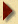3rd Puzzle Answer

To calculate the probability that at least 2 people among 40 have the same birthday , it is simpler to calculate first the probability that no one has the same birthday. The total number of combinations is 365^40 (we ignore leap years, if one is born on the 29th of Feb, one presumably celebrates one's birthday on the 1st of March 3 times out of 4).
There are 365x364x363x....x326 ways of choosing 40 people's birthdays so that they are all different = 365! / (365-40)!
The probability that no one has the same birthday is: 365!/ [325! x 365^40] = 0.109
And the odds are 89.1 % in favour of your friend. To make the bet fair the odds should be roughly 9 to 1. Change friend !Puzzle Page